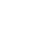4:1衰减法控制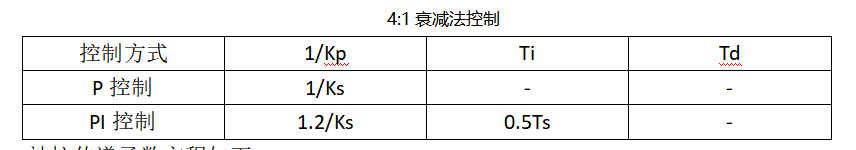Gp(s) =1 / 100^3 + 80^s + 17s + -1;

两个峰值之间的时间周期 Ts = 21.9967；

P控制：比例系数 Kp = Ks = 4.74；

PI控制：比例系数 Kp = Ks/1.2 = 3.95；

积分时间常数 Ti = 0.5 * Ts = 10.9984;

积分系数 Ki = Kp / Ti = 3.95 / 10.9984 = 0.3591;

P（比例）控制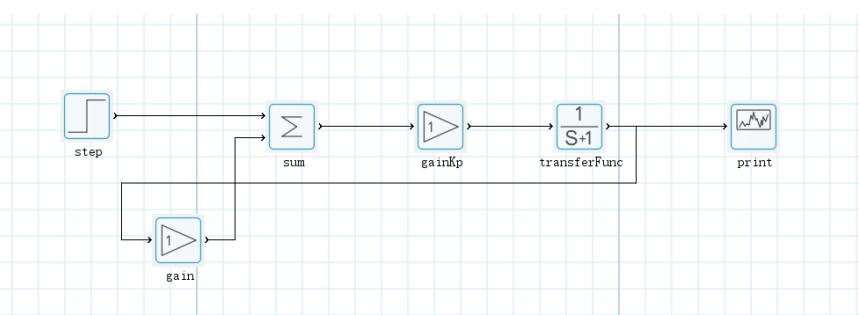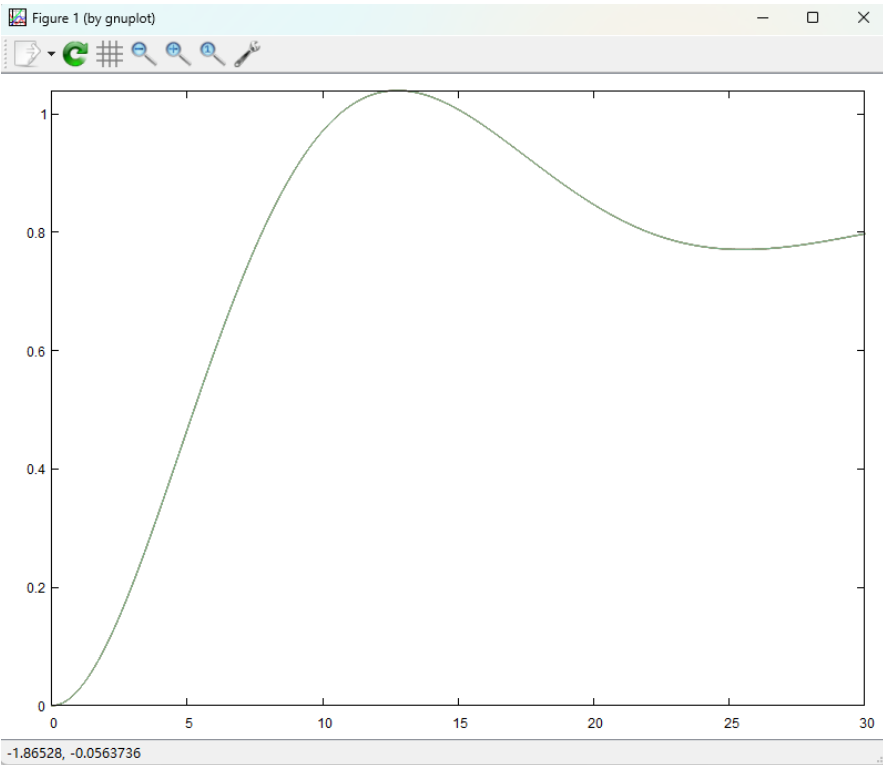PI（比例积分）控制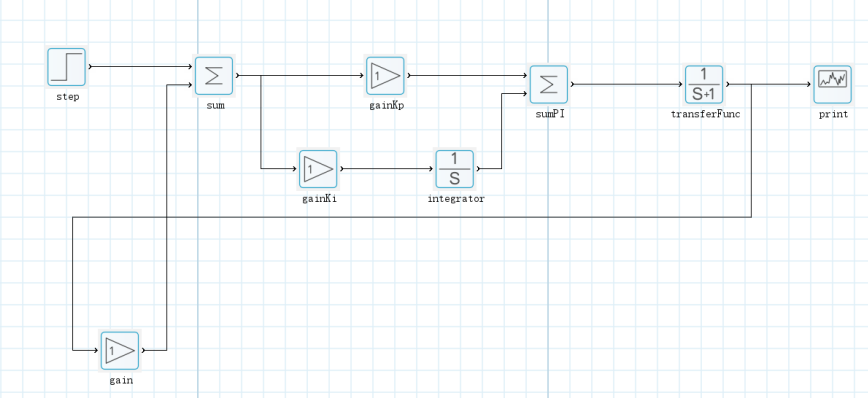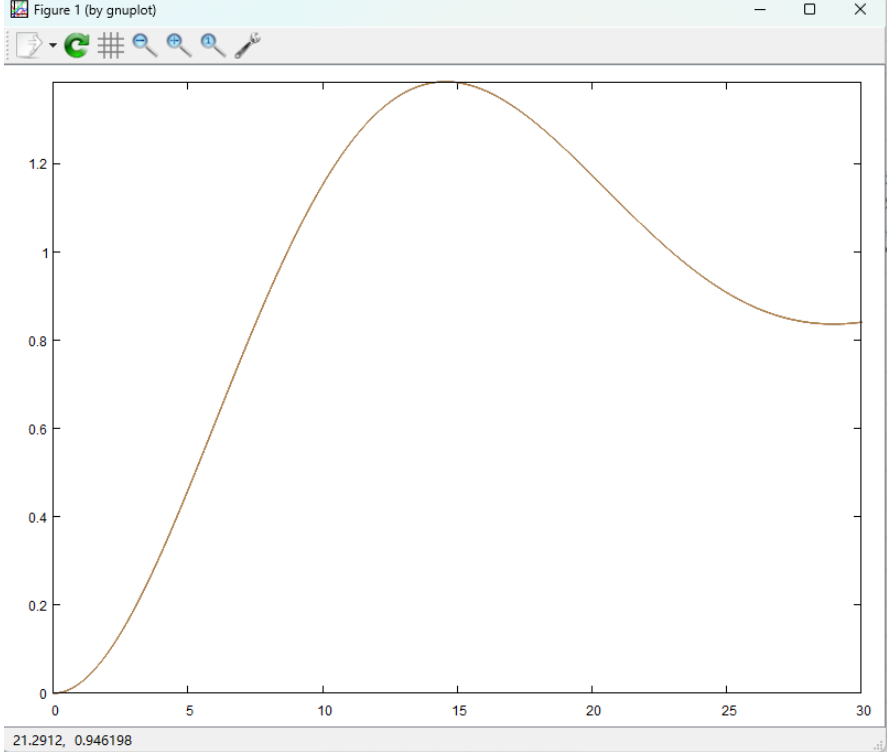## 回复

### 社区牛人